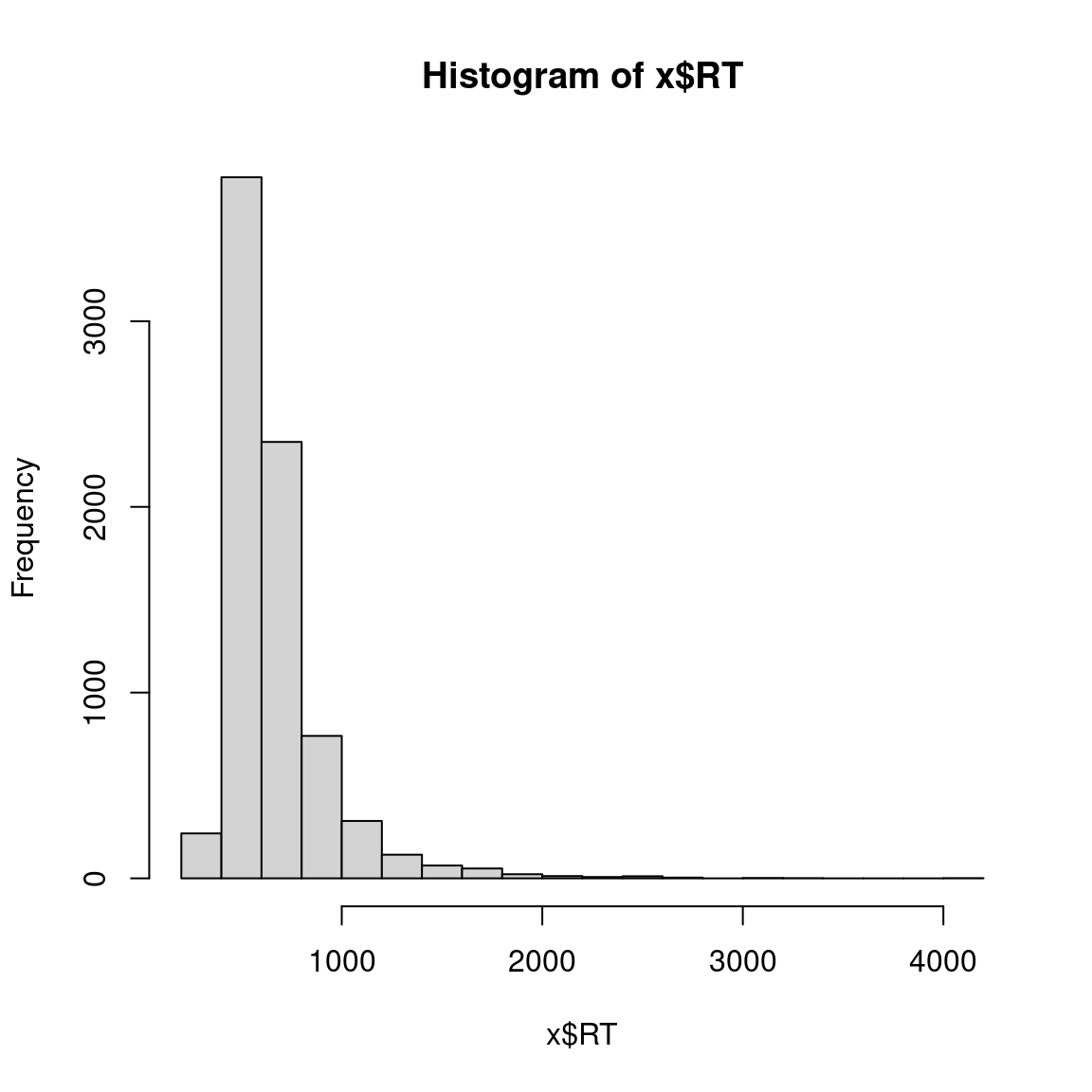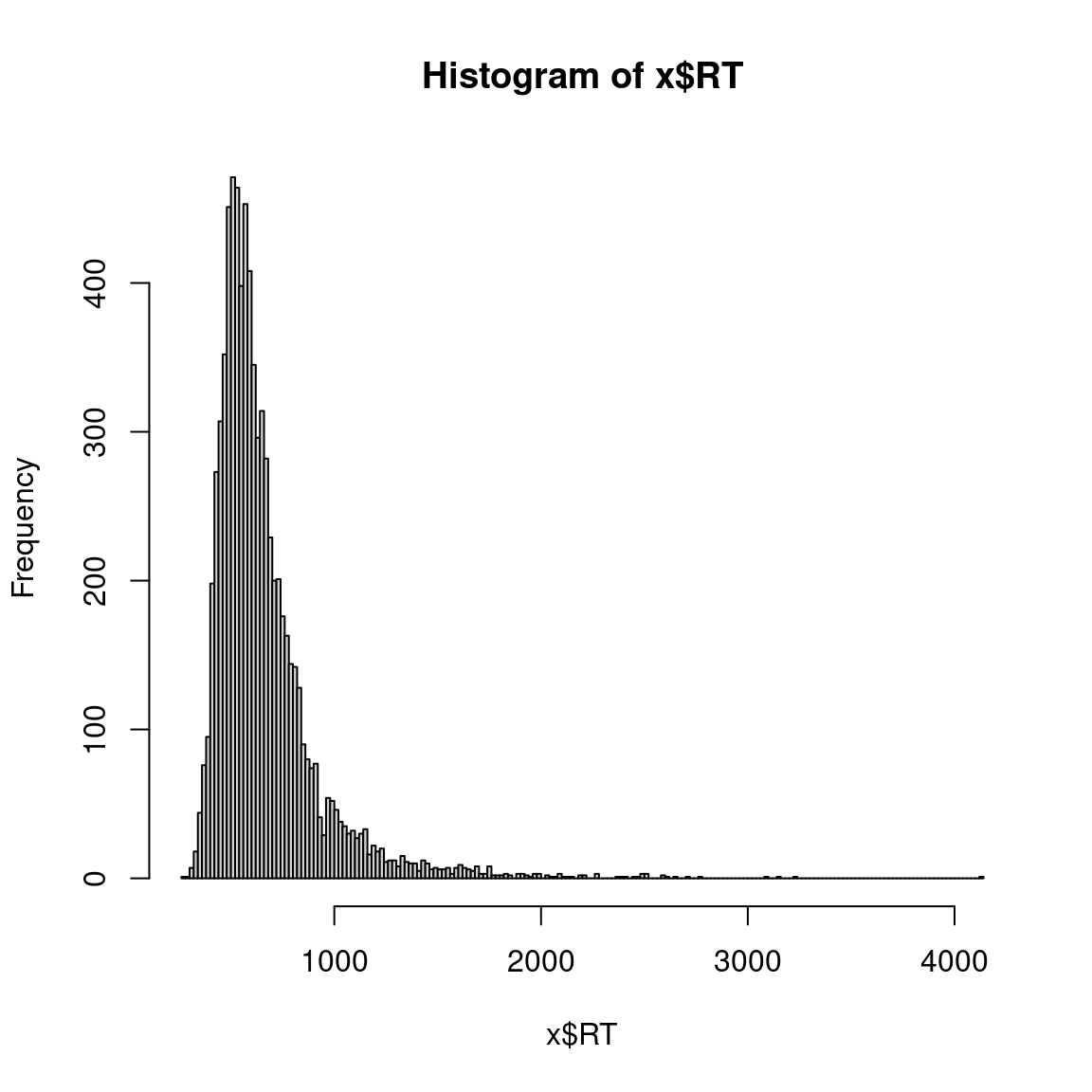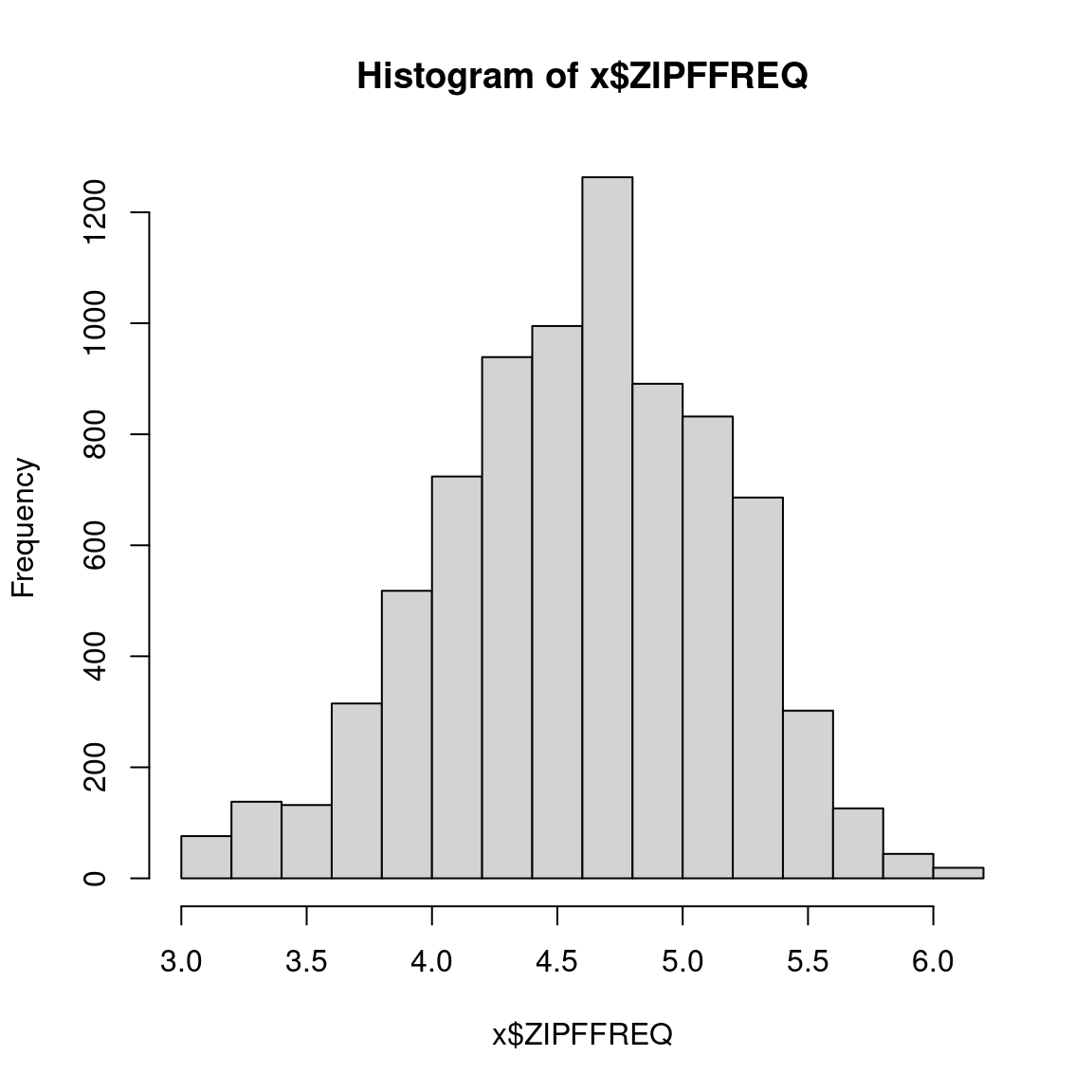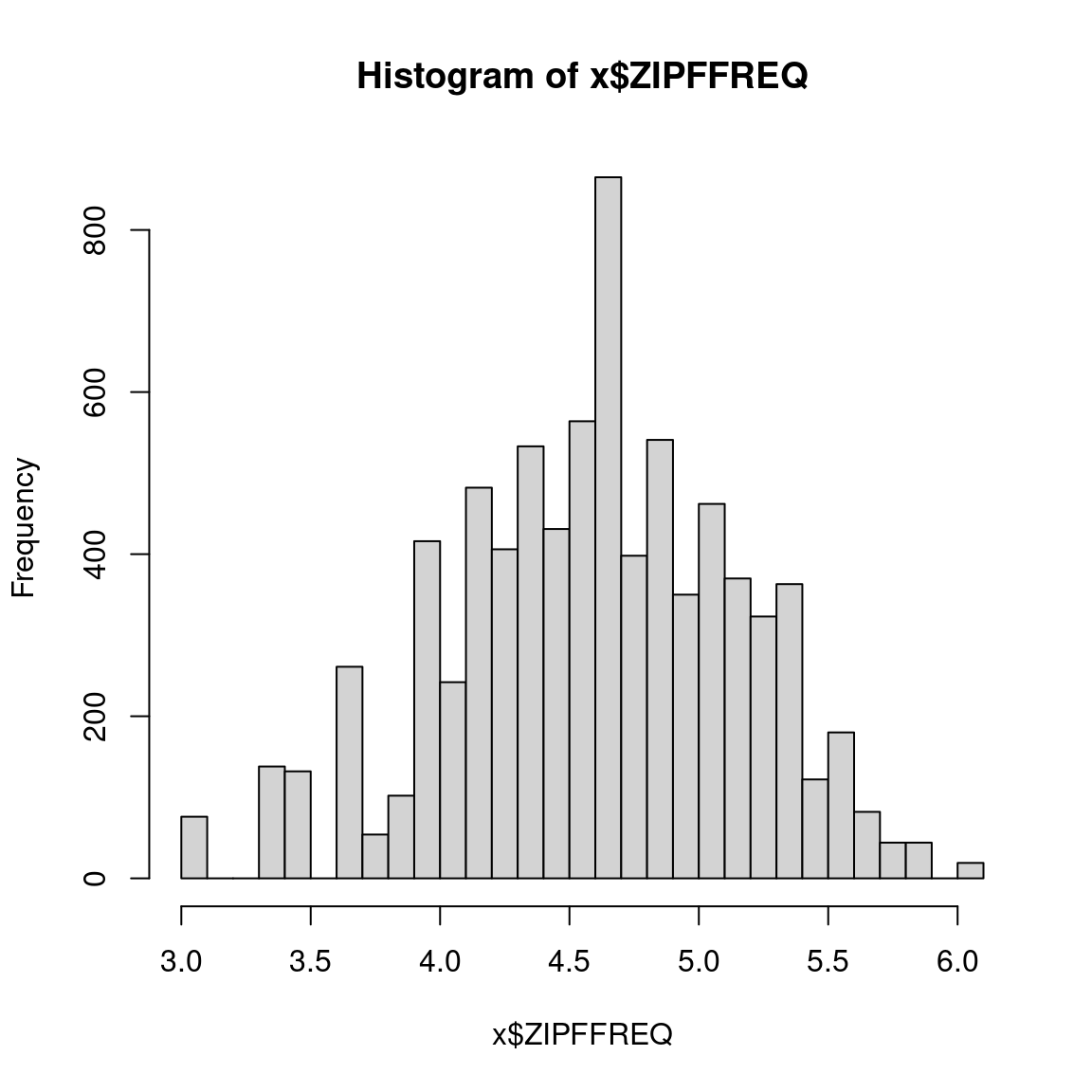# 1 Session 03: Linear modeling 2

Discussion of SFLWR3, Section 5.5

## 1.1 Class activities

### 1.1.1 A multifactorial model selection process

Does the reaction time to a word (in ms) vary as a function of

• the Zipf frequency of that word (`ZIPFFREQ`);
• the language that word was presented in (`LANGUAGE`: English vs. Spanish);
• the speaker group that words was presented to (`GROUP`: English vs. heritage);
• any pairwise interaction of these predictors;
• the three-way interaction of these predictors?
``````rm(list=ls(all.names=TRUE))
library(car); library(effects)
file="105_02-03_RTs.csv",  # this file
stringsAsFactors=TRUE)) # change categorical variables into factors``````
``````##       CASE            RT             LENGTH      LANGUAGE        GROUP
##  Min.   :   1   Min.   : 271.0   Min.   :3.000   eng:4023   english :3961
##  1st Qu.:2150   1st Qu.: 505.0   1st Qu.:4.000   spa:3977   heritage:4039
##  Median :4310   Median : 595.0   Median :5.000
##  Mean   :4303   Mean   : 661.5   Mean   :5.198
##  3rd Qu.:6450   3rd Qu.: 732.0   3rd Qu.:6.000
##  Max.   :8610   Max.   :4130.0   Max.   :9.000
##                 NA's   :248
##       CORRECT        FREQPMW           ZIPFFREQ     SITE
##  correct  :7749   Min.   :   1.00   Min.   :3.000   a:2403
##  incorrect: 251   1st Qu.:  17.00   1st Qu.:4.230   b:2815
##                   Median :  42.00   Median :4.623   c:2782
##                   Mean   :  81.14   Mean   :4.591
##                   3rd Qu.: 101.00   3rd Qu.:5.004
##                   Max.   :1152.00   Max.   :6.061
## ``````

Some exploration of the relevant variables:

``````# the predictor(s)/response on its/their own
hist(x\$RT); hist(x\$RT, breaks="FD")````````hist(x\$ZIPFFREQ); hist(x\$ZIPFFREQ, breaks="FD")````table(x\$LANGUAGE)``
``````##
##  eng  spa
## 4023 3977``````
``table(x\$GROUP)``
``````##
##  english heritage
##     3961     4039``````
``````# the predictor(s) w/ the response
plot(
main="RT in ms as a function of frequency per million words",
pch=16, col="#00000030",
xlab="Zipf frequency", xlim=c(  3,    6), x=x\$ZIPFFREQ,
ylab="RT (in ms)"    , ylim=c(250, 4250), y=x\$RT); grid()
abline(lm(x\$RT ~ x\$ZIPFFREQ), col="red", lwd=2)``````